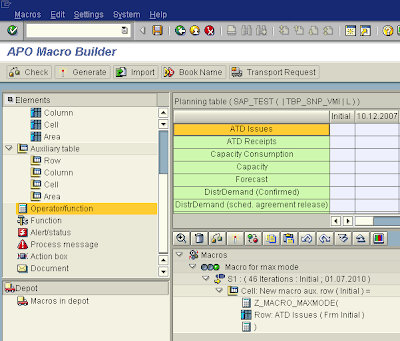## Wednesday, February 20, 2008

### Tutorial on ABAP functions for DP macros

It seems that there is no standard function to calculate the statistical mode. So I thought it would make a good example for a short tutorial on creation of ABAP functions to be used in planning macros.

The macro functions are standard functions created with SE37 that must have a specific call signature (input and output parameters and tables). The base parameters are the ones shown in the following figure (later other options are presented).The VALUE_TAB structure has two fields, one for numbers and one for strings. All input parameters set in the macro are added to this table in the same order as defined in the macro, in the number or string field depending on the data type.

For our exemple the function will receive a list of numbers. The goal is to calculate the mode of the integer part of the numbers. Since the mode can be a list of several numbers, we intend to return the largest integer number of the list.

Bellow is the code for such a function. It just counts the frequency of the numbers (after convertion to integers). The final list is sorted and the mode (or maximum of modes) is returned on the output parameter field F_ARGUMENT. The other parameter F_CALC_ERROR is a flag that should be set in case of errors that cannot be handled (the equivalent of raising an exception).

`FUNCTION z_macro_maxmode .*"----------------------------------------------------------------------*"*"Local interface:*"  TABLES*"      VALUE_TAB STRUCTURE  /SAPAPO/VALUE_TAB*"  CHANGING*"     REFERENCE(F_CALC_ERROR) TYPE  C*"     REFERENCE(F_ARGUMENT) TYPE  /SAPAPO/MXSOP-V*"---------------------------------------------------------------------- TYPES:   BEGIN OF t_histogram,     value   TYPE i,     counter TYPE i,   END OF t_histogram. DATA:   lv_value TYPE i,   ls_hist  TYPE t_histogram,   lt_hist  TYPE TABLE OF t_histogram WITH KEY value. LOOP AT value_tab.   IF value_tab-string IS INITIAL.     CLEAR ls_hist.*     just truncated integers to make it simpler     lv_value = trunc( value_tab-value ).     READ TABLE lt_hist WITH TABLE KEY value = lv_value INTO ls_hist.     IF sy-subrc = 0.*     already in the table, increase the counter       ls_hist-counter = ls_hist-counter + 1.       MODIFY TABLE lt_hist FROM ls_hist TRANSPORTING counter.     ELSE.*     insert value for the first time in the table       ls_hist-value = lv_value.       ls_hist-counter = 1.       INSERT ls_hist INTO TABLE lt_hist.     ENDIF.   ENDIF. ENDLOOP.* return the integer with largest counter (if more than one return the* largest integer) SORT lt_hist BY counter DESCENDING value DESCENDING. READ TABLE lt_hist INDEX 1 INTO ls_hist. f_argument = ls_hist-value.ENDFUNCTION.`

Now this function can be added to the macro builder (/sapapo/advm). First one registers the function using the following menu option.A list of parameters is shown. The previous macro only uses the base parameters so no other checkbox is flagged, but here is the place to choose the parameter options.Then the macro is created with the new function being called like the standard ones.I've found that moving logic to ABAP functions can be a good way to simplify complex macros.

Labels: , ,